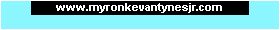Question:
How do I Make a Negative Number Positive in Game Maker?In an earlier question, we talked about how to make positive numbers negative. Well how do you make a negative number positive? Do the same thing as before!

Let's say we want to make a variable positive. Our variable is called myDirection and it equals -5:

 myDirection=-5;

Any number multiplied by 1 gives you the same number, and multiplying a negative number by a negative number gives you a positive number! So here's the code:

 myDirection=myDirection*-1;

This code multiplies the value of myDirection by -1, and then puts the answer back in the myDirection variable. Now myDirection is positive! Nice!

 Page updated: March 23rd, 2015 @ 6:21 AM Eastern Time

 Our other websites: